## Table of ContentOpen Access

ARTICLE

# Fast Segmentation Method of Sonar Images for Jacket Installation Environment

Hande Mao1,2, Hongzhe Yan1, Lei Lin1, Wentao Dong1,3, Yuhang Li1, Yuliang Liu2,4,*, Jing Xue5

1 COOEC-Fluor Heavy Industries Co., Ltd., Zhuhai, 519000, China
2 Zhejiang Ocean University, Zhoushan, 316022, China
3 Saint Petersburg State Marine Technical University, Saint Petersburg, 190000, Russia
4 Wenzhou Institute of Technology, Wenzhou, 325035, China
5 Deakin University, Burwood, VIC 3125, 430070, Australia

* Corresponding Author: Yuliang Liu. Email:Intelligent Automation & Soft Computing 2023, 36(2), 1671-1686. https://doi.org/10.32604/iasc.2023.028819

## Abstract

It has remained a hard nut for years to segment sonar images of jacket installation environment, most of which are noisy images with inevitable blur after noise reduction. For the purpose of solutions to this problem, a fast segmentation algorithm is proposed on the basis of the gray value characteristics of sonar images. This algorithm is endowed with the advantage in no need of segmentation thresholds. To realize this goal, we follow the undermentioned steps: first, calculate the gray matrix of the fuzzy image background. After adjusting the gray value, the image is divided into three regions: background region, buffer region and target regions. After filtering, we reset the pixels with gray value lower than 255 to binarize images and eliminate most artifacts. Finally, the remaining noise is removed by morphological processing. The simulation results of several sonar images show that the algorithm can segment the fuzzy sonar images quickly and effectively. Thus, the stable and feasible method is testified.

## Keywords

1  Introduction

Jacket is a kind of indispensable equipment for ocean development. And most sonar images of jacket installation environment are noisyimages with inevitable blur after noise reduction. The noise generated by the marine environment brings great difficulties in segmentation and recognition of sonar images. There are few references on how to segment the blurred sonar images after noise reduction. The Markov random field studied by Ali et al. [1,2] has a good effect on segmentation of sonar images. Xiang et al.  devised the method of maximum entropy segmentation to improve the segmentation speed. Li et al.  modify the particle swarm optimization method and achieved good results. Today, some existing methods spend a lot of time in threshold calculation [10,11] such as Otsu method  and iterative threshold method [15,16]. Although these methods are widely used, their results are unstable. Our ideal result is to get the binary image which only contains the target. Therefore, this paper proposes a new method, which segments the sonar images according to the characteristics of the gray value of them. Only the pixels with gray value lower than 255 are reset to complete the preliminary segmentation. Firstly, the background gray matrix of the image is calculated. Second, the background region, buffer region and target region are divided by the mapping equation. In order to prevent too many artifacts in subsequent operations, we need to ensure that the fluctuation range of gray value is very small in the same region. After that, the gray value of the whole image is adjusted. After above steps, the gray value of the target is 255. At this time, the gray value of pixels less than 255 can be changed to 0, and the purpose of preliminary segmentation can be achieved by removing redundant artifacts. Finally, segmentation is completed by morphological image processing. In this segmentation process, it is not necessary to calculate the segmentation threshold, so as to achieve the purpose of fast image segmentation. The simulation of multiple sonar images by MATLB shows that this method is feasible and stable.

2  Model of Noise Images

As shown in Fig. 1, in order to verify the stability and feasibility of the process, the following three sonar images are selected as the research objects. Due to the influence of equipment performance, seabed suspended solids and other factors, sonar images will be greatly disturbed in the imaging process. The above problems bring great difficulties to the segmentation of sonar images.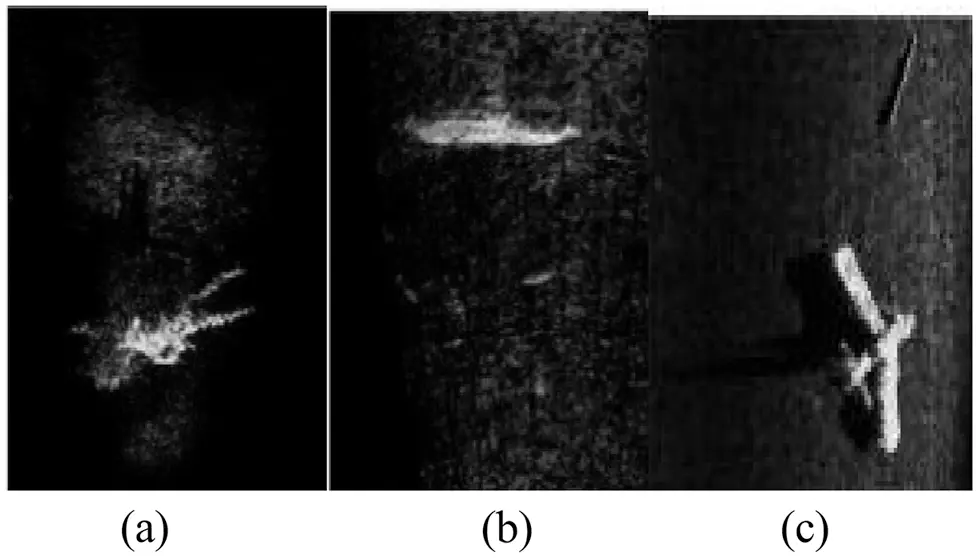Figure 1: Sample images: (a) Frogman and bubble. (b) Fish. (c) Aircraft on the seabed

In the process of signal transmission [17,18], due to the complex underwater environment,  most images contain noise. In order to verify the feasibility of the algorithm, it is necessary to apply noise to the sample images  according to the actual underwater noise. After noise reduction, those images are segmented to ensure the accuracy of the results.

First of all, the underwater noises and those impact on the image need to be analyzed. There are three kinds of underwater noise: reverberation of active sonar, sea ambient noise and sonar self-noise, in which the sea ambient noise appears as Gaussian noise and is inevitably disturbed during the underwater imaging. The reverberation of active sonar is speckle noise, especially in the shallow water.

The amplitude of the sea ambient noise obeys Gaussian distribution, and its model  is as follows:

ϕ(z)=1σ2πe(zμ)2σ2, (1)

in which, z stands for the gray value of the image. The mean value is as follows:

μ=+ϕ(z)zdz, (2)

Its variance is as follows:

σ2=+(zμ2)ϕ(z)dz, (3)

As shown in Fig. 2, Gaussian noise with mean value of 0 and variance of 0.03 is added to sonar image respectively.Figure 2: Images with Gaussian noise

Next, we will consider the reverberation model of active sonar. The reverberation noise in the images is shown as multiplicative speckle noise, and its model is as follows:

N(i,j)=O(i,j)n(i,j), (4)

in which, N (i, j) represents the amplitude of the noise pixel at point (i, j). O (i, j) is the original image, and n (i, j) is the noise that the original image suffers. In consideration of few denoising methods for multiplicative noise, it is required to approximate it to additive noise to facilitate image processing. Its formula is as follows:

N(i,j)=n¯O(i,j)+O¯(n(i,j)n¯), (5)

in which, n¯ is the average value of noise and its value is constant 1.

As shown in Fig. 3, the speckle noise with standard deviation of 1.2 is added to the samples.Figure 3: Images with speckle noise

These two kinds of noise are inevitable to some extent. Therefore, sonar images need to be denoised . Next, we need to denoise those images, and design the corresponding process to segment them. By means of the segmentation result comparison between of proposed method and other methods, the stability and feasibility of the algorithm are verified.

3  Selection of Noise Reduction Methods and Design of Algorithm Processes

3.1 The Processing of Sonar Images by Guided Filtering

Guided filtering  has a good effect on sonar image denoising. It is applied to calculate the relationship between near points and target points by virtue of the locally linear model. Suppose I and q respectively stand for the input image and output image, and K represents the center pixel of mask Wk and then their locally linear relationship is as follows:

qi=akIi+bi,iWk, (6)

in which, the mask center is K. a and b are constant coefficients.

The linear relationship between the input image and the guide image is ▽ q = aI, which ensures that the output image have gradient. The relationship between the input noise image (p) and the output image (q) is qi = pini. In order to minimize the difference between the input image and the output image, and calculate the coefficients a and b, the following results can be obtained:

E(ak,bk)=iwk((akIi+bkpi)2)+iiwkϵak2, (7)

After solving, the values of a and b can be obtained as follows:

{ak=1|w|iwkIipiμkp¯kσk2+ϵbk=p¯akμk, (8)

in which, |w| is the total number of pixels in the mask, σk2 is signifies the variance of the guide image I within the mask wk, and μk symbolizes the mean value of the guide image I in the mask wk.

Each pixel is contained by multiple linear masks. The output value of them are equal to the average value of the pixels in multiple linear masks, with its calculation formula as follows:

qi=k:iwk(akIi+bk)=a¯iIi+b¯i, (9)

The values of ai and bi are obtained as follows:

{a¯i=1|w|kwiakb¯i=1|w|kwibk, (10)

As shown in Fig. 4, guided filtering is used to filter sonar images interfered by Gaussian noise.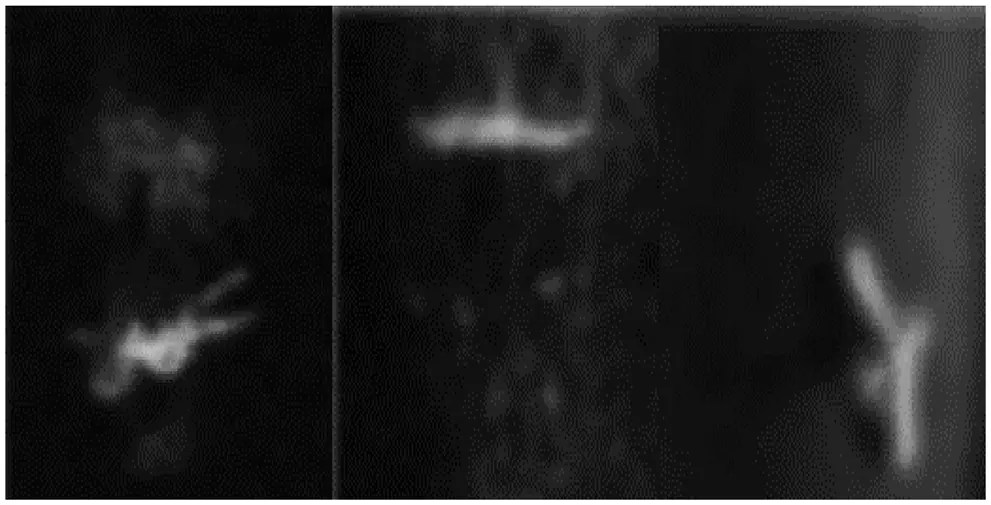Figure 4: Result of processing Gaussian noise by guided image filter

The guided filter is used for processing of multiple sonar images, and most of the Gaussian noise in the images has been removed. This shows that the guided filter has a good effect on the Gaussian noise caused by sea ambient noise.

Next, the effect of guided filtering on speckle noise caused by reverberation of active sonar is tested. Fig. 5 implies that the guided filter is applied to deal with the speckle noise in those images.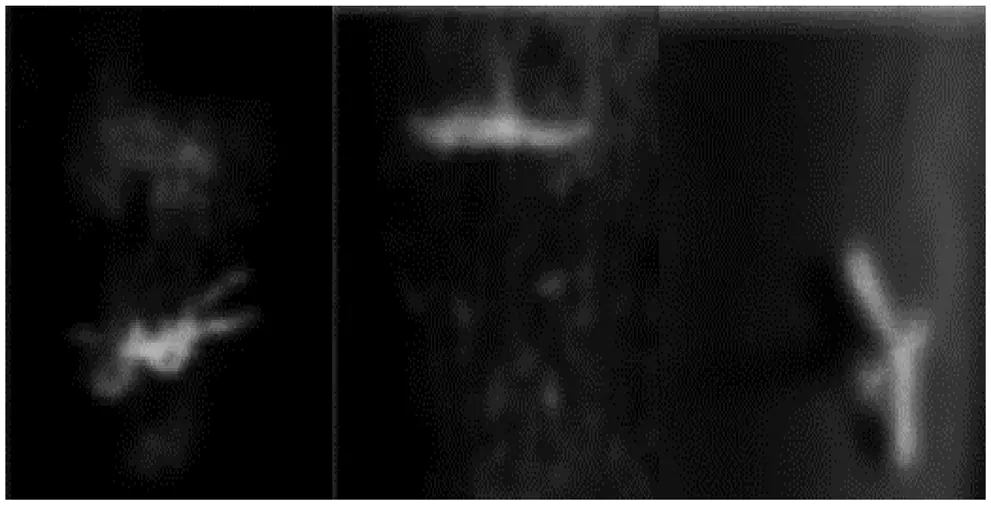Figure 5: Result of processing speckle noise by guided image filter

After the guided filter is used for samples, it is found that most of the noise in images has been removed. It is shown that the guided filtering has a good effect on the speckle noise caused by reverberation of active sonar.

In consideration of the whole images blurred by noise reduction, however, it is necessary to segment the samples under the condition of the integrity of the target form.

3.2 Design of Processing

As shown in Fig. 6, the gray histograms of sonar images are needed to grasp general distribution features of the gray value. According to them, we can design the corresponding segmentation process.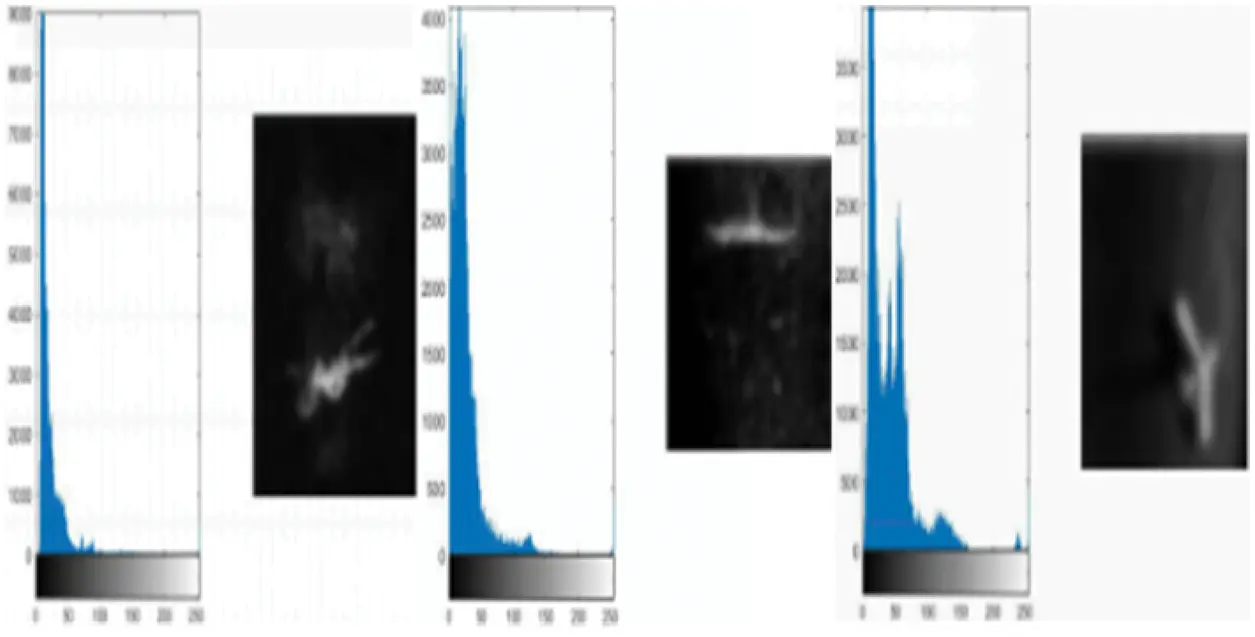Figure 6: Gray histogram of sonar image after noise reduction

Judging from the traversal and statistic data of gray histograms, the gray value of target far greater than that of the background. The background gray value is largely about 0.1 * 255; likewise, the pixels around the target show their gray value higher than that of the peripheral background. Thus, the sonar image is divided into three obvious regions. The process could be devised as Fig. 7, with reference to characteristics mentioned above.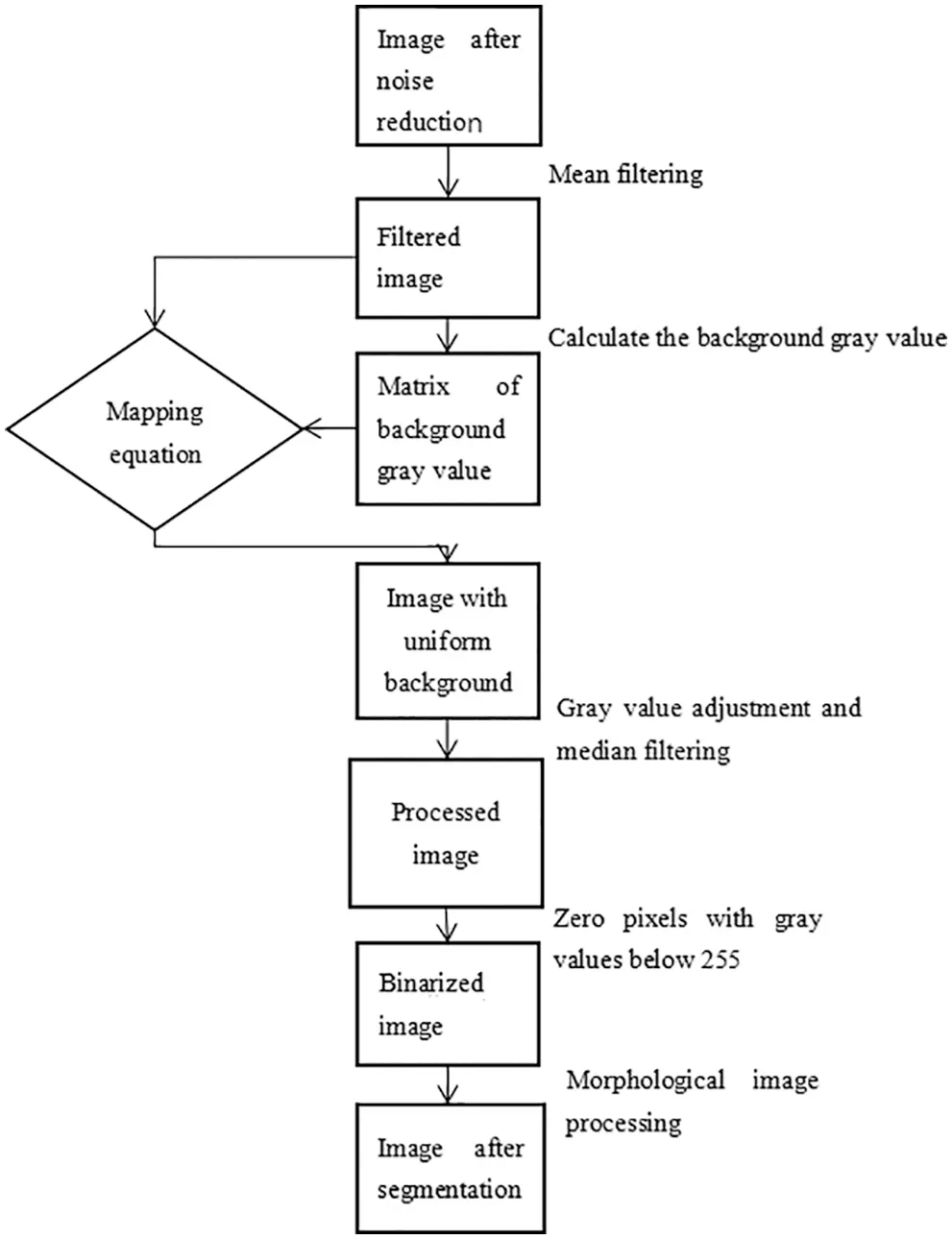Figure 7: Segmentation process of sonar image segmentation

As elicited from the flow chart, for image segmentation, the background gray value matrix needs to be calculated.

4  Preliminary Image Segmentation

4.1 Calculation of Background Gray Value Matrix

It is imperative to calculate the background gray value matrix of the denoised image, for the purpose of segmentation and repair such incomplete parts of the target such as bubbles, head and legs of the frogman. First, use a 9 * 9 mask to filter the image. The mean filtering can lower down the fluctuation amplitude of the image gray value and raise the gray value around the main object in the image and thus, laying the foundation for the calculation of the background gray value matrix.

Then, we finalize the mask size according to the target size in the image. To do so, we use a 7 * 7 mask to traverse all the pixels in the image. We pick out and record n points with the highest gray values among the 49 points centered on g (x, y). Next, we need to sort the recorded gray values in a descending order to form a set M. In consideration of the interference of residual noise in the image, remove both maximum and minimum gray values before calculating the background gray value. Then, we calculate the average value of the remaining gray values in the set as the background gray value of the mask center point g (x, y). The calculated background gray value matrix and its histogram are shown in Fig. 8.Figure 8: Background gray value matrix and its histogram

After the background gray value is calculated, the mapping equation is used to adjust the gray value of the whole image to the characteristics of underwater acoustic image gray value. Then, the image is divided into three regions in the ascending order of the gray value, namely background region, buffer region and target region.

4.2 Mapping of Image Gray Value

Next, the mapping equation is applied to map the background matrix onto the original image for adjustment of the gray value. The background gray value at point (x, y) is B, the gray value of the original image at (x, y) is A, and the gray value at point (x, y) after adjustment is E. The gray value is adjusted as steps indicate in Fig. 9.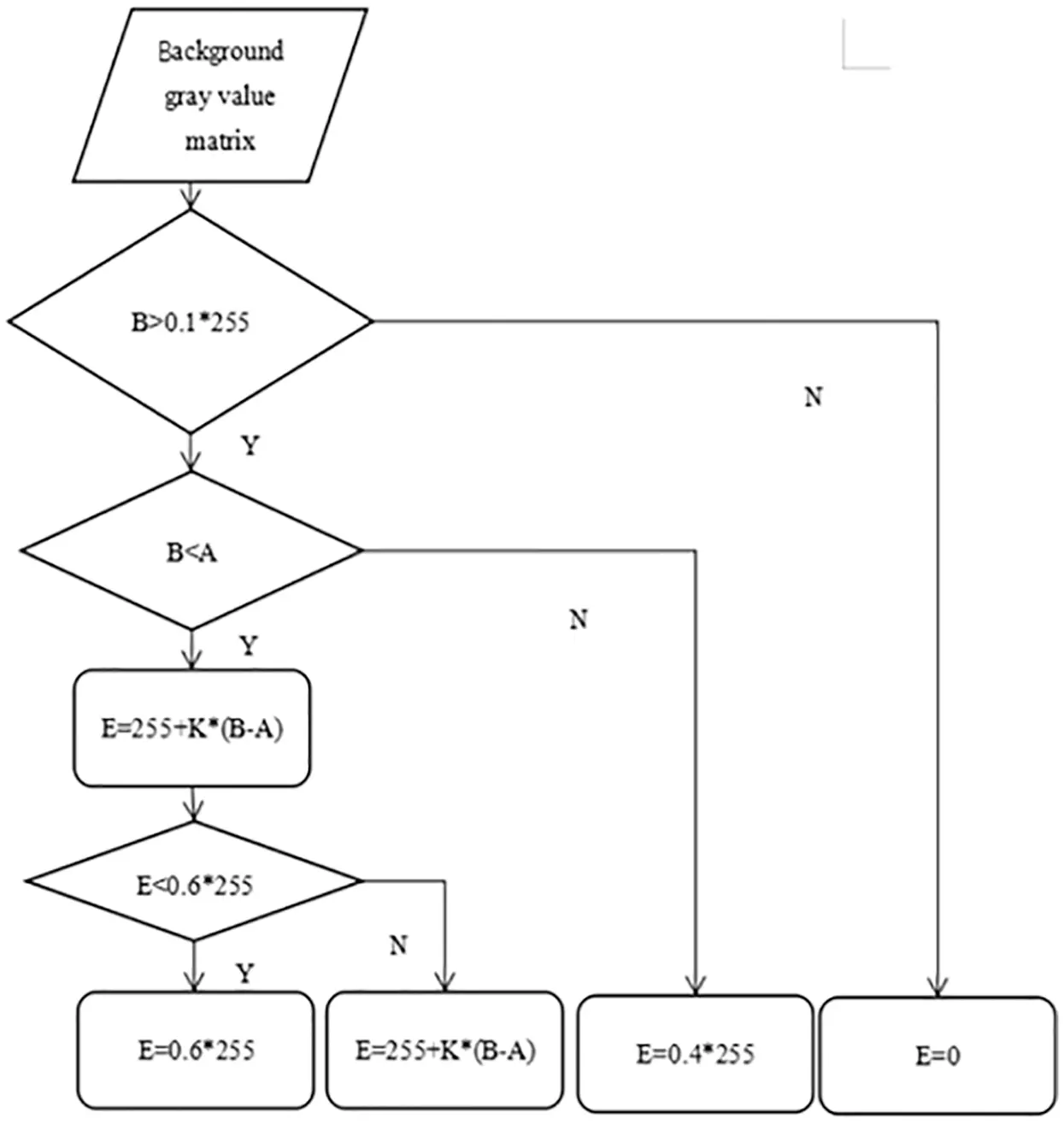Figure 9: Mapping equation

According to the gray histogram, when the gray value B in the background matrix is lower than 0.1 * 255, the point is likely to be the background region. If the point appears in the target region, because it can be compensated by median filter in subsequent processing, its gray value can be directly set to 0.

Since the image has undergone the mean filter before the gray value adjustment, the point is likely to be the target when B is less than A, so it is to ascertain whether E is less than 0.6 * 255 after adjustment. If E is lower than 0.6 * 255, it may indicate that the gray value of this part of the image is overshadowed by that of other parts in the target region, so the gray value of this part of the image is increased to 0.6 * 255.

If E is greater than 0.6 * 255, it is the interior of the target region, so it is necessary to suppress the gray value of this point, so as to lay a foundation for raising the overall gray value of the target to 255.

Finally, if B is greater than A, it means that the probability of this point is probably to be around the target region or to be the target region. Therefore, set its gray value as 0.4 * 255 to ensure that the following compensation with median filtering will not impose excessive impacts upon the target shape.

When K acts as the magnification factor. Due to differences in the background gray value, the multiplication of subtraction is also different. And we want to make this image create artifacts in the low gray value area of the target and suppress the area with high gray value in the target area, so as to lay a foundation for the overall gray value of the target to reach 255 after gray stretching. K is valued as follows:

{K=5B220K=(3E60)/120100<B<220K=222.5B100 (11)

The mapped images are shown in Fig. 10.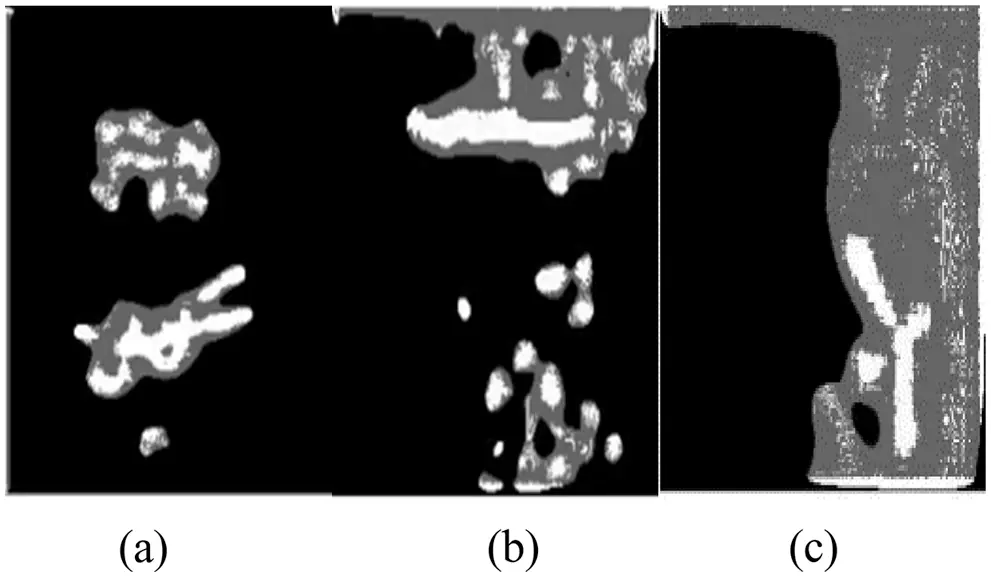Figure 10: Mapped images: (a) Frogman and bubble. (b) Fish. (c) Aircraft on the seabed

According to the gray value, the images are divided into three regions: background region, buffer region and target region. At this time, the pixels in the target region of the images are still incoherent, so median filtering is needed. The buffer region ensures that the target area will not be interfered excessively after median filtering.

Due to the target size in the sonar image, select a 3 * 3 mask for median filtering. After median filtering, stretch the gray scale or adopt Retinex [25,26] algorithm to enhance those images:

r(x,y)=kKwk{logS(x,y)log[FK(x,y)S(x,y)]} (12)

in which, r (x, y) is the output image, S (x, y) is the original image, and K is the number of functions. At this time, K = 3, and W1 = W2 = W3 = 1/3.

The preliminary segmentation results are shown in Fig. 11.Figure 11: Preliminary segmentation results

Obviously, the fracture in the target region is repaired, and the interference pixels in the buffer region are also reduced. At this time, the gray values of the target region are 255.

Next, it is necessary to reset the pixels with gray values below 255 and then segment the sonar images through processing of such pixels in a morphological way.

5  Simulation Results

The gray values of the target in the images are 255. The rest parts of background region and buffer region are the background parts after segmentation. As shown in Fig. 12, fulfill binarization by resetting pixels with gray values less than 255.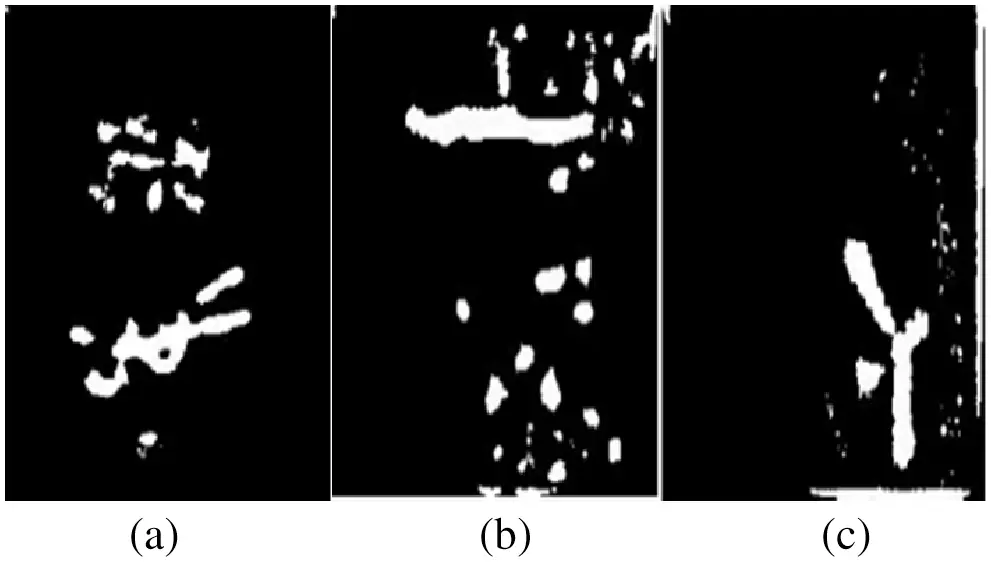Figure 12: Images after binarization: (a) Binary image of frogman and bubble. (b) Binary image of fish. (c) Binary image of aircraft on the seabed

After binary, some artifacts are eliminated. And the remaining artifacts are used to repair the target. The region with low gray value is enhanced, which is convenient for the following morphological processing of the images.

For the binarized sample that is acquired, the steps are taken as follows to carry out morphological processing necessary for segmentation: First, define a 4 * 4 square structure element B. After erosion algorithm, the small target removal algorithm is performed on the result image to remove the area less than 150 pixels. Then, for the sake of spindle-shaped underwater objects, a flat structure element C with radius of 4 is defined to perform the dilation algorithm on the image. As shown in Fig. 13, a binarized sample is obtained, with only targets contained in the sample.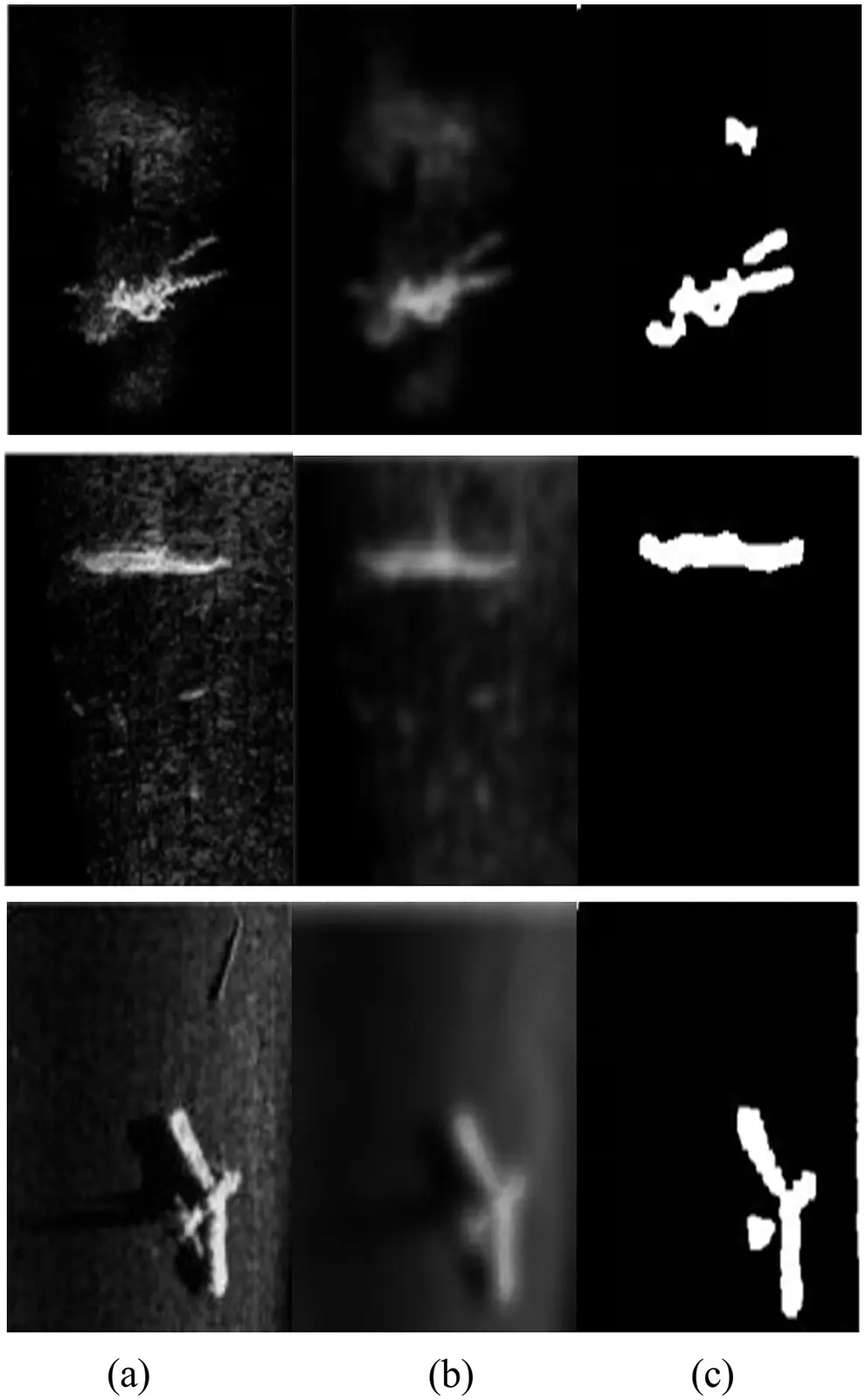Figure 13: Segmentation results of the method in this paper: (a) Original images. (b) Blurred images after noise reduction. (c) Segmentation results

As shown in Fig. 14, if the main target is the frogman, it is feasible to eliminate the bubble by morphological dilation and erosion and small target removal algorithm. And duration of proposed method is shown in Tab. 1.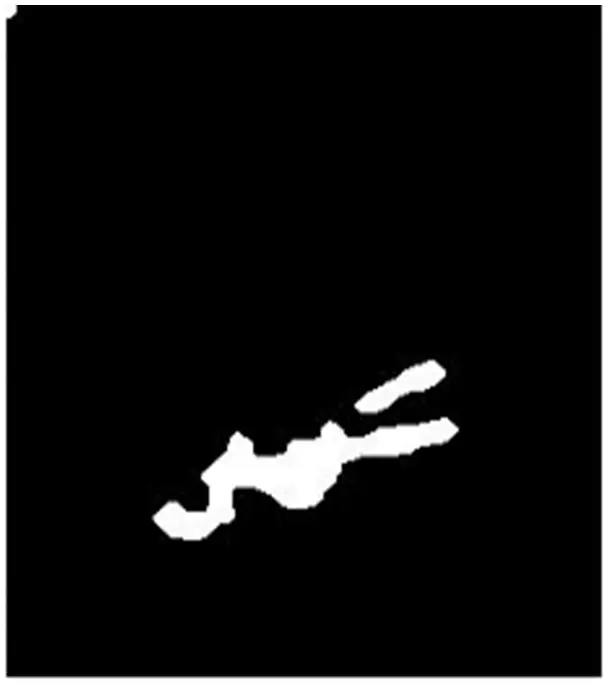Figure 14: Image with bubbles removed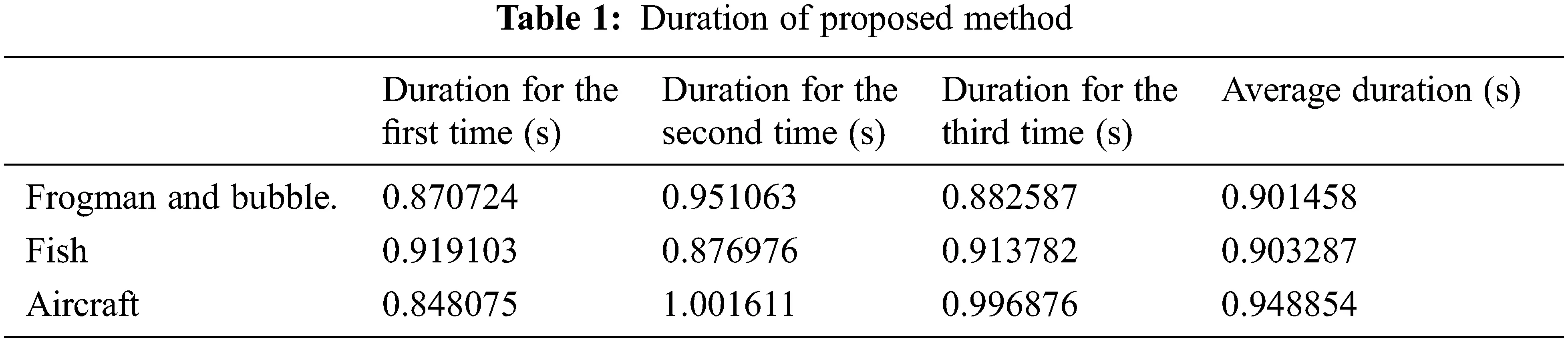Finally, the method adopted in this study is compared with other segmentation methods, such as Otsu method, one-dimensional maximum entropy segmentation method and iterative threshold method in terms of segmentation time and segmentation effect. As shown in Fig. 15, Otsu method aims at segmentation based on the binarization threshold of the images. And duration of Otsu method is shown in Tab. 2.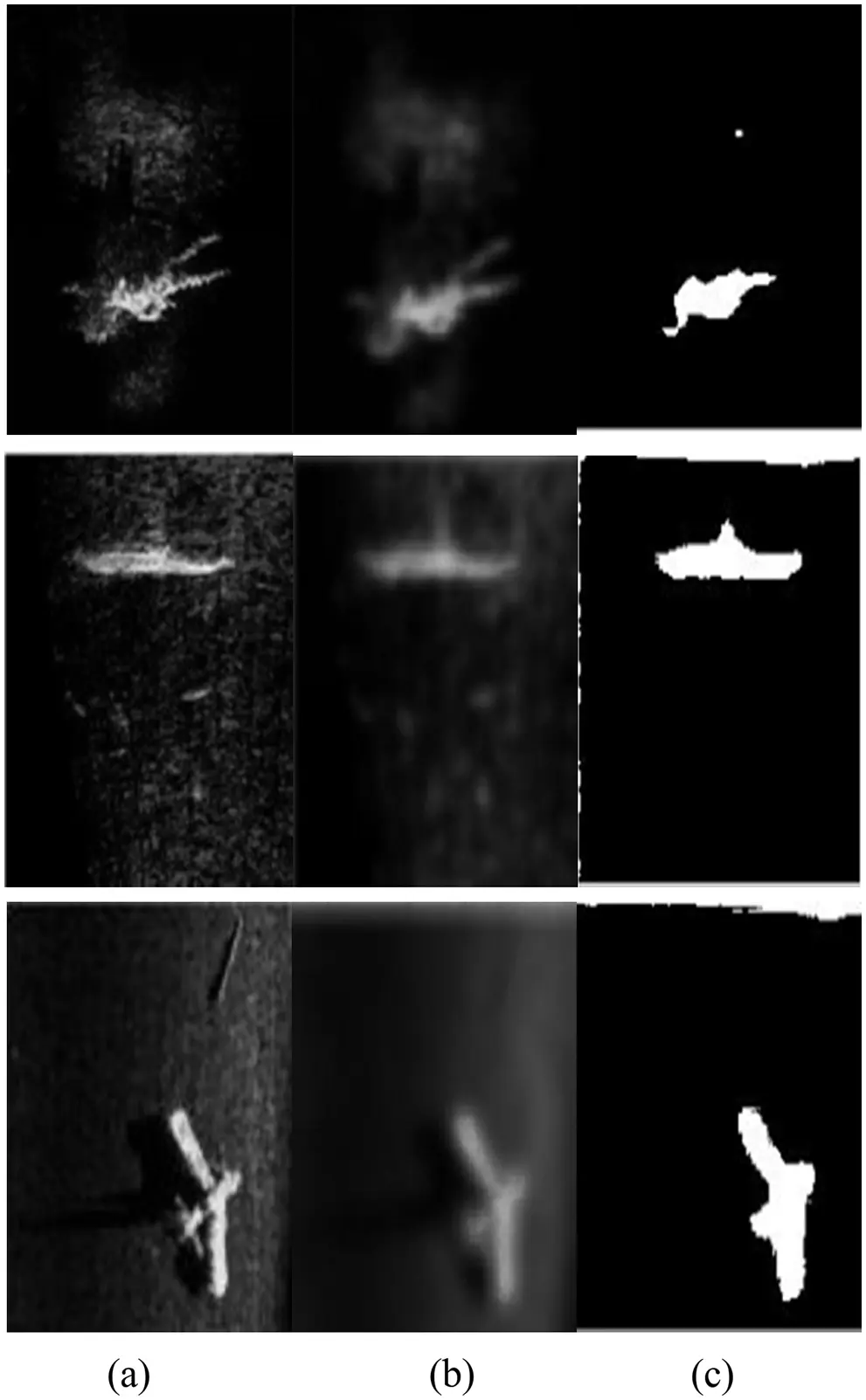Figure 15: Segmentation results of Otsu: (a) Original images. (b) Blurred images after noise reduction. (c) Segmentation results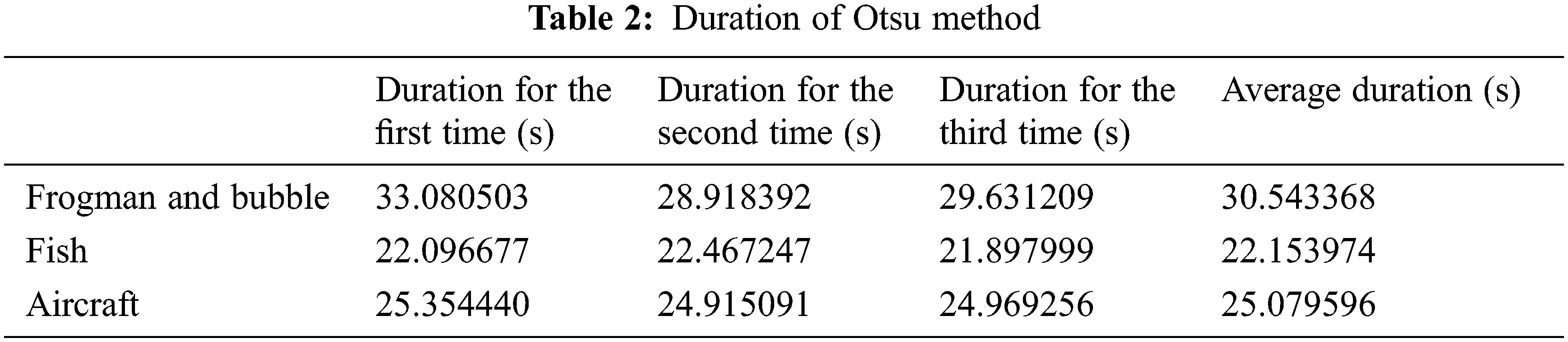As shown in Fig. 16, the entropy in the images represents the average amount of information, and the threshold T is determined with reference to the entropy after one-dimensional maximum entropy segmentation method is adopted. And duration of this method is shown in Tab. 3.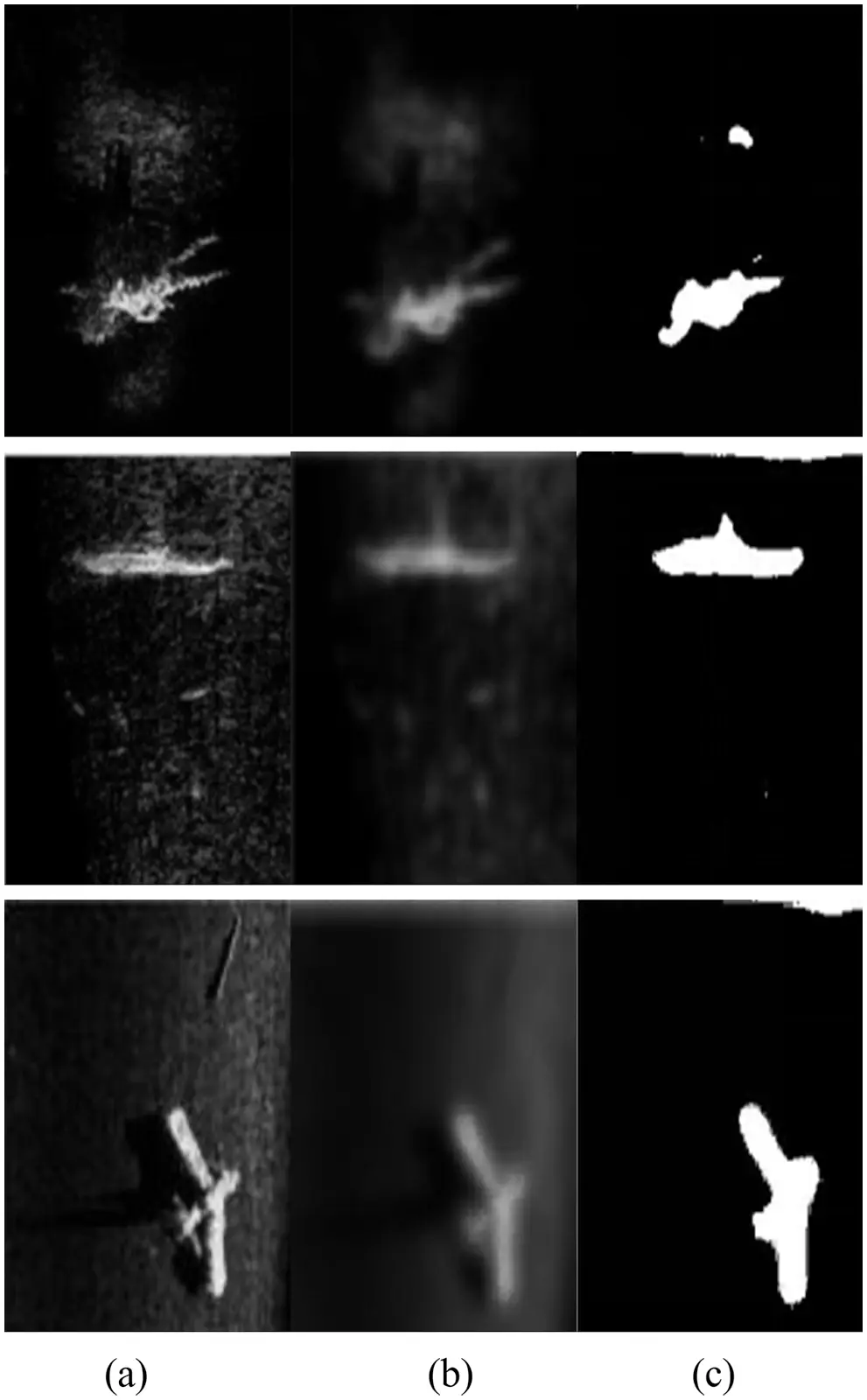Figure 16: Segmentation results of maximum entropy method: (a) Original images. (b) Blurred images after noise reduction. (c) Segmentation results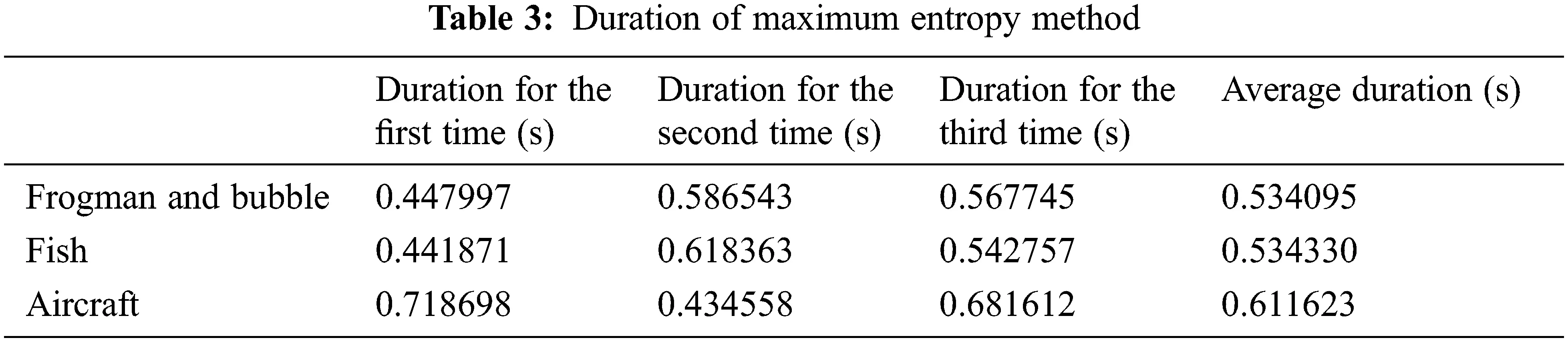As shown in Fig. 17, iterative threshold method is taken mainly to select the threshold. And duration of this method is shown in Tab. 4.Figure 17: Segmentation results of Iterative threshold method: (a) Original images. (b) Blurred images after noise reduction. (c) Segmentation results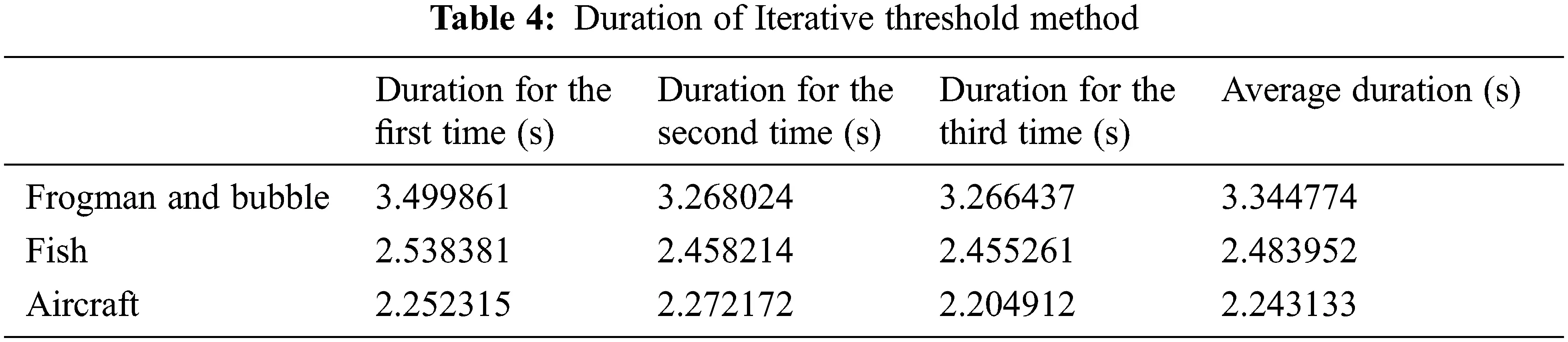The evaluation of the algorithms  from two aspects of segmentation effect  and segmentation time discloses that: after segmentation with adoption of this method, the samples have the relatively intact morphology, the target shows a full shape for the high-quality segmentation from the images. This is conspicuous in the segmentation of frogman and bubble image; from the perspective of execution time, the proposed method does not take so long because of no need in calculation of the segmentation threshold, and thus, the execution time for different images doesn’t fluctuate bitterly.

From two aspects of segmentation result and segmentation time, it is proved that the method is feasible and stable.

6  Conclusion

In this paper, in order to solve the image segmentation problem of jacket installation environment, a fast segmentation algorithm for blurred sonar images is proposed, and the simulation results prove that the algorithm applies the precise segmentation of sonar images. Before segmentation, three kinds of noises which may affect the sonar images are taken into consideration: reverberation of active sonar, sea ambient noise and sonar self-noise. After that, the blurred sonar images are obtained by eliminating the above noise by guided filtering.

During the preliminary segmentation of sonar images, such steps as filtering, background gray value matrix calculation and gray value adjustment were taken to preliminarily segment multiple sonar images. Finally, the blurred sonar images were segmented by virtue of morphological processing after the image was binarized directly without calculation of the threshold value.

Finally, this method is proved to be stable and feasible.

Funding Statement: This work was supported by Open Fund Project of China Key Laboratory of Submarine Geoscience (KLSG1802), Science & Technology Project of China Ocean Mineral Resources Research and Development Association (DY135-N1-1-05), and Science & Technology Project of Zhoushan city of Zhejiang Province (2019C42271, 2019C33205).

Conflicts of Interest: The authors declare that they have no conflicts of interest to report regarding the present study.

## References

1. A. Ali and D. M. Reza, “Improving the runtime of MRF based method for MRI brain segmentation,” Applied Mathematics and Computation, vol. 2015, no. 1, pp. 808–818, 2015.
2. S. M. Choi, J. E. Lee, J. Kim and M. H. Kim, “Volumetric object reconstruction using the 3D-MRF model-based segmentation [magnetic resonance imaging],” IEEE Trans. Medical Imaging, vol. 16, no. 6, pp. 887–892, 1997.
3. Q. Xiang, L. Peng and X. Pang, “Image DAEs based on residual entropy maximum,” IET Image Processing, vol. 14, no. 6, pp. 1164–1169, 2020.
4. Y. Li, Z. Li, Z. Ding, T. Qin and W. Xiong, “Automatic infrared ship target segmentation based on structure tensor and maximum histogram entropy,” IEEE Access, vol. 8, pp. 44798–44820, 2020.
5. Y. Xu, S. Hu and Y. Du, “Bias correction of multiple MRI images based on an improved nonparametric maximum likelihood method,” IEEE Access, vol. 7, pp. 166762–166775, 2019.
6. T. Li, X. Tang and Y. Pang, “Underwater image segmentation based on improved PSO and fuzzy entropy,” The Ocean Engineering, vol. 28, no. 2, pp. 128–133, 2010.
7. H. Lee, N. C. F. Codella, M. D. Cham, J. W. Weinsaft and Y. Wang, “Automatic left ventricle segmentation using iterative thresholding and an active contour model with adaptation on short-axis cardiac MRI,” IEEE Trans., Biomedical Engineering, vol. 57, no. 4, pp. 905–913, 2010.
8. J. Li, J. Zhou, Y. Chen, F. Han and Q. H. Liu, “Retrieval of composite model parameters for 3-D microwave imaging of biaxial objects by BCGS-FFT and PSO,” IEEE Trans. Microwave Theory and Techniques, vol. 68, no. 5, pp. 1896–1907, 2020.
9. Y. Xue, A. Aouari, R. F. Mansour and S. Su, “A hybrid algorithm based on PSO and GA for feature selection,” Journal of Cyber Security, vol. 3, no. 2, pp. 117–124, 2021.
10. A. M. Wan. “A proposed optimum threshold level for document image binarization,” Advanced Research in Computing and Applications, vol. 7, no. 1, pp. 8–14, 2017.
11. H. Jaafar, D. A. Ramli and S. Ibrahim. “A robust and fast computation touchless palm print recognition system using LHEAT and the IFKNCN classifier,” Computational Intelligence and Neuroscience, vol. 2015, no. 5, pp. 1–17. 2015.
12. A. K. Bhandari, I. V. Kumar and K. Srinivas, “Cuttlefish algorithm-based multilevel 3-D otsu function for color image segmentation,” IEEE Trans. Instrumentation and Measurement, vol. 69, no. 5, pp. 1871–1880, 2020.
13. A. K. Khambampati, D. Liu, S. K. Konki and K. Y. Kim, “An automatic detection of the ROI using Otsu thresholding in nonlinear difference EIT imaging,” IEEE Sensors Journal, vol. 18, no. 12, pp. 5133–5142, 2018.
14. B. Chen, X. Zhang, R. Wang, Z. Li and W. Deng, “Detect concrete cracks based on Otsu algorithm with differential image,” The Journal of Engineering, vol. 2019, no. 23, pp. 9088–9091, 2019.
15. S. Roychowdhury, D. D. Koozekanani and K. K. Parhi, “Iterative vessel segmentation of fundus images,” IEEE Trans. Biomedical Engineering, vol. 62, no. 7, pp. 1738–1749, 20
16. L. Yu, Y. Zhang, Q. Zhang, Y. Ji and Z. Dong, “Minimum-entropy autofocusing based on re-PSO for ionospheric scintillation mitigation in P-band SAR imaging,” IEEE Access, vol. 7, pp. 84580–84590, 2019.
17. W. Zhuang, Y. Chen, J. Su, B. Wang and C. Gao, “Design of human activity recognition algorithms based on a single wearable IMU sensor,” International Journal of Sensor Networks, vol. 30, no. 3, pp. 193–206, 2019.
18. W. Zhuang, Y. Shen, L. Li, C. Gao and D. Dai, “Develop an adaptive real-time indoor intrusion detection system based on empirical analysis of OFDM subcarriers,” Sensors, vol. 21, no. 7, pp. 2287–2287, 2021.
19. J. Zhou, “Research on denoising method of underwater acoustic image,” M.S. dissertation, University of Electronic Science and Technology, Chengdu, 20
20. Z. A. and A. M. Aly, “Natural convection in an H-shaped porous enclosure filled with a nanofluid,” Computers, Materials & Continua, vol. 66, no. 3, pp. 3233–3251, 2021.
21. Y. Li and X. Wang, “Person re-identification based on joint loss and multiple attention mechanism,” Intelligent Automation & Soft Computing, vol. 30, no. 2, pp. 563–573, 20
22. Y. Yang, W. Wan, S. Huang, F. Yuan, S. Yang et al., “Remote sensing image fusion based on adaptive IHS and multiscale guided filter,” IEEE Access, vol. 4, pp. 4573–4582, 2016.
23. X. Luo, H. Zeng, Y. Wan, X. Zhang, Y. Du et al., “Endoscopic vision augmentation using multiscale bilateral-weighted retinex for robotic surgery,” IEEE Trans. Medical Imaging, vol. 38, no. 12, pp. 2863–2874, 2019.
24. Z. Li and J. Zheng, “Single image de-hazing using globally guided image filtering,” IEEE Trans. Image Processing, vol. 27, no. 1, pp. 442–450, 2018.
25. M. Lecca, “STAR: A segmentation-based approximation of point-based sampling milano retinex for color image enhancement,” IEEE Trans. Image Processing, vol. 27, no. 12, pp. 5802–5812, 2018.
26. Y. Pu, “A fractional-order variational framework for retinex: Fractional-order partial differential equation-based formulation for multi-scale nonlocal contrast enhancement with texture preserving,” IEEE Trans. Image Processing, vol. 27, no. 3, pp. 1214–1229, 2018.
27. X. Guo, Y. Li, J. Ma and H. Ling, “Mutually guided image filtering,” IEEE Trans. Pattern Anal. and Mach. Intell, vol. 42, no. 3, pp. 694–707, 2020.
28. S. S. Alrumiah and A. A. Al-Shargabi,“Educational videos subtitles’ summarization using latent dirichlet allocation and length enhancement,” Computers, Materials & Continua, vol. 70, no. 3, pp. 6205–6221, 2022.
29. Z. Zhang and T. Kang, “Prediction model of abutment pressure affected by far-field hard stratum based on elastic foundation theory,” Computers, Materials & Continua, vol. 66, no. 1, pp. 341–357, 2021.
30. X. R. Zhang, W. F. Zhang, W. Sun, X. M. Sun and S. K. Jha, “A robust 3-D medical watermarking based on wavelet transform for data protection,” Computer Systems Science & Engineering, vol. 41, no. 3, pp. 1043–1056, 2022.

H. Mao, H. Yan, L. Lin, W. Dong, Y. Li et al., "Fast segmentation method of sonar images for jacket installation environment," Intelligent Automation & Soft Computing, vol. 36, no.2, pp. 1671–1686, 2023.This work is licensed under a Creative Commons Attribution 4.0 International License , which permits unrestricted use, distribution, and reproduction in any medium, provided the original work is properly cited.

View

Like

## Related articles

• Yuxiang Huang, Chuliu He, Jiaqiu...
• Ang Li, Guo-jian Shao, Pei-rong...
• Yuchun Duan, Xiaoming Zhang, Yuqing...
• Stephen R. Niezgoda, Surya R....
• W.C. Wang, F.L. Chang, Y.L. Liu,...